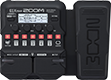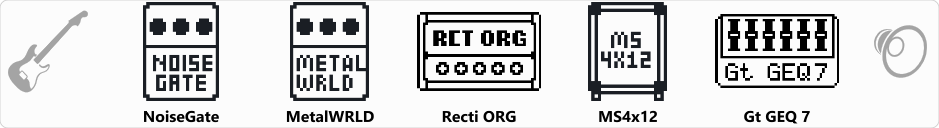# BEST METAL DIST

Discussion in 'Zoom G1/G1X Four' started by pedro felicio, Jun 25, 2023.

1. BEST METAL DISTDevice: Zoom G1X Four
Firmware: 2.00

Name on device: BEST METAL
Optimized for: Phones/Speaker

Effects chain:best metal dist

Effect: "NoiseGate" (Dynamics), active - "yes"
"Detect" = GTRIN
"Depth" = 80
"Threshold" = 30
"Decay" = 0

Effect: "MetalWRLD" (Overdrive / Distortion), active - "yes"
"Gain" = 25
"Bass" = 60
"Treble" = 65
"Volume" = 60

Effect: "Recti ORG" (Amp simulator), active - "yes"
"Mode" = MDRN
"Bass" = 20
"Middle" = 50
"Treble" = 70
"Presence" = 30
"Gain" = 50
"Volume" = 15

Effect: "MS4x12" (Cabinet), active - "yes"
"MIC" = ON
"D57: D421" = 75
"Hi" = 65
"Lo" = 40

Effect: "Gt GEQ 7" (Filter), active - "yes"
"100" = -4.0
"200" = -4.0
"400" = -1.5
"800" = -12.0
"1.6k" = 5.5
"3.2k" = 0.0
"6.4k" = 12.0
"VOL" = 65

Patch Volume: 100